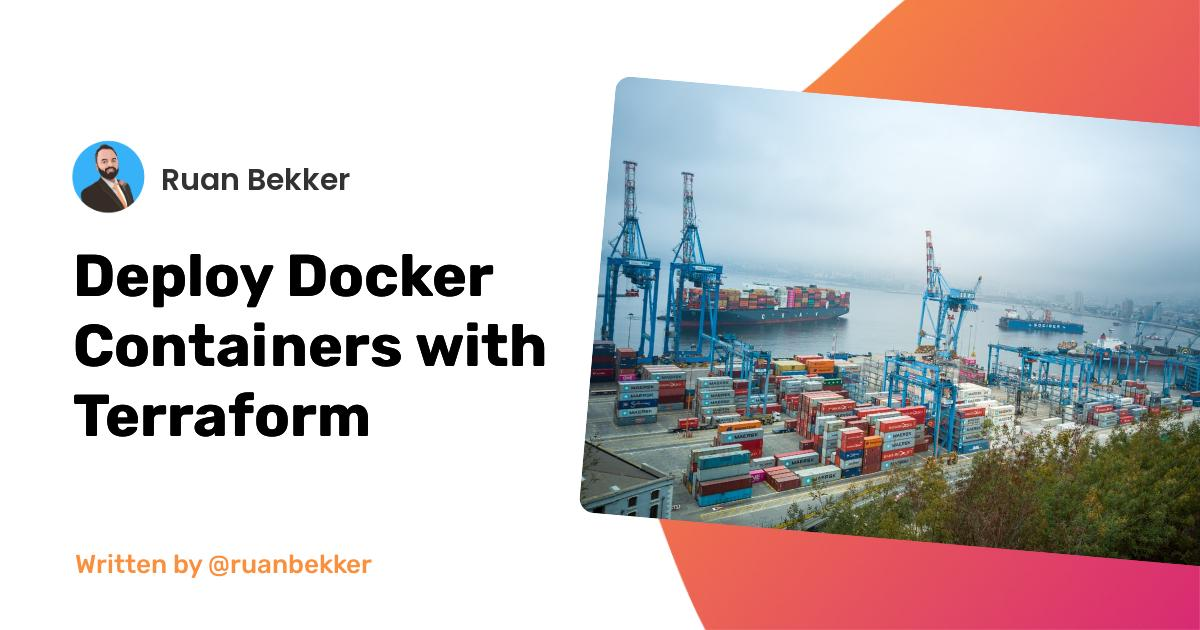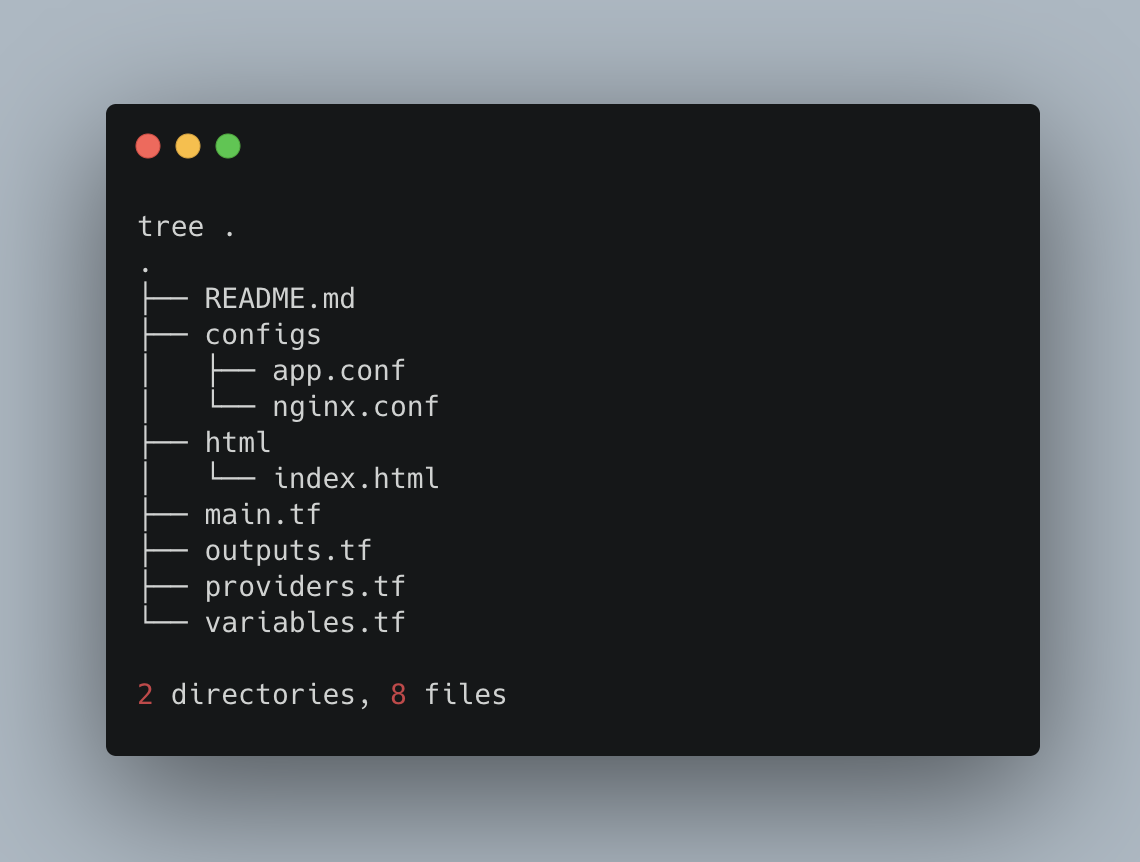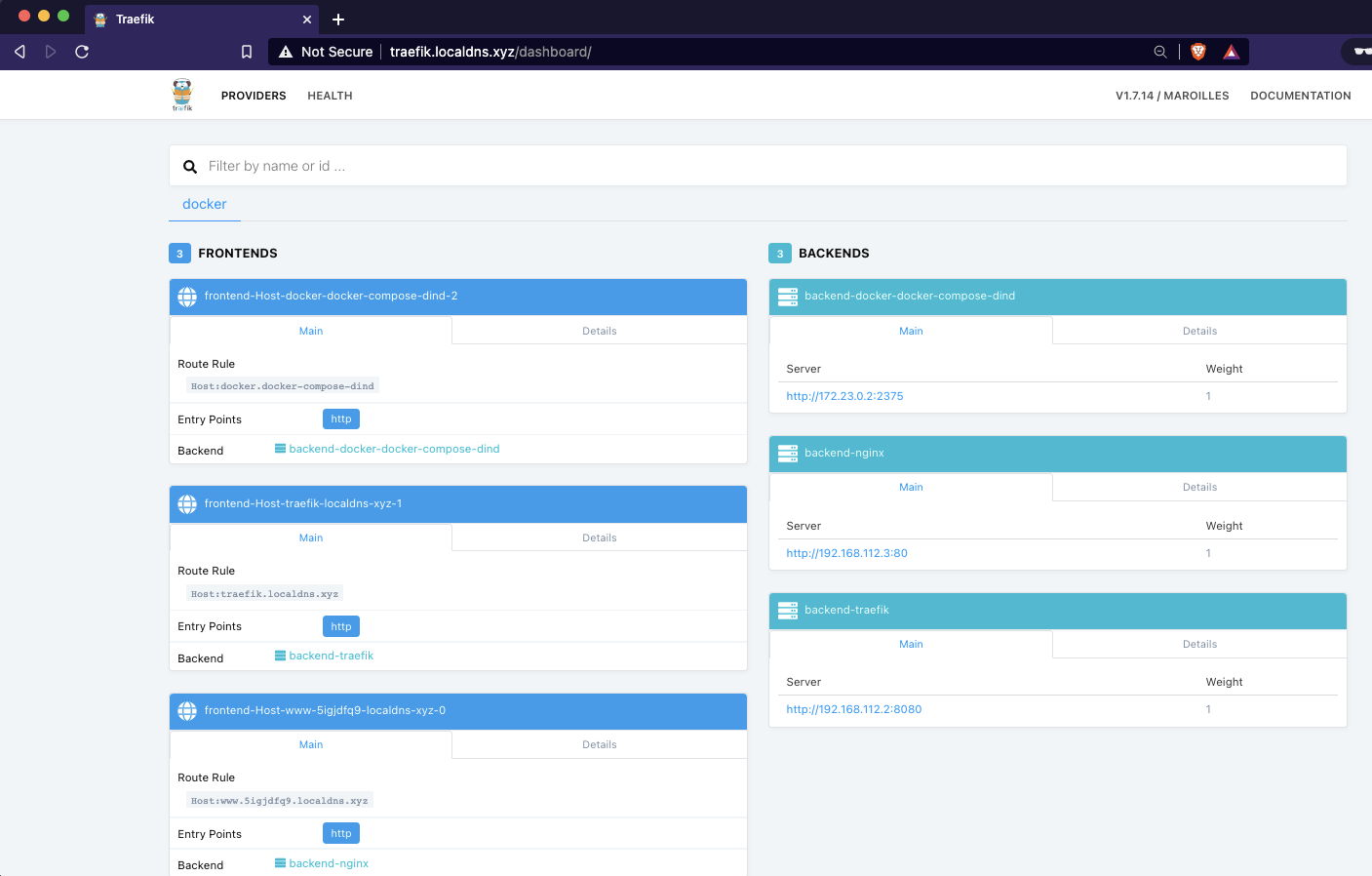# Deploy Docker Containers with Terraform

Written by Ruan Bekker on Dec 5th, 2021 Views

In this post I will demonstrate how to use the terraform docker_container resource from the docker provider to run two docker containers, traefik and nginx and use the random provider to generate a random url for us.

## Pre-Requisites

You will require terraform and docker to be installed.

## Project Structure

The source code for this post is available on my github repository, but the project structure will look like the following:Our `providers.tf`:

``````terraform {
required_providers {
docker = {
source  = "kreuzwerker/docker"
version = "2.15.0"
}
random = {
version = "~> 3.0"
}
}
}

provider "docker" {
host = "unix:///var/run/docker.sock"
}

provider "random" {}
``````

Our `variables.tf`:

``````variable "domain" {
type    = string
default = "localdns.xyz"
}
``````

Our `outputs.tf`:

``````output "nginx_container_name" {
value = docker_container.nginx.name
}

output "traefik_container_name" {
value = docker_container.traefik.name
}

output "traefik_url" {
value = "http://traefik.\${var.domain}/"
}

output "nginx_url" {
value = "http://www.\${random_string.nginx.result}.\${var.domain}/"
}
``````

Our `main.tf`:

``````resource "random_string" "nginx" {
length  = 8
upper   = false
special = false
}

resource "docker_image" "nginx" {
name = "nginx:stable-alpine"
}

resource "docker_image" "traefik" {
name = "traefik:1.7.14"
}

resource "docker_network" "nginx" {
name   = "docknet"
driver = "bridge"
}

resource "docker_container" "traefik" {
name  = "traefik"
image = docker_image.traefik.name

name    = docker_network.nginx.name
aliases = ["docknet"]
}

restart = "unless-stopped"
destroy_grace_seconds = 30
must_run = true
memory = 256

volumes {
host_path      = "/var/run/docker.sock"
container_path = "/var/run/docker.sock"
}

command = [
"--api",
"--docker",
"--docker.watch",
"--logLevel=INFO"
]

ports {
internal = 80
external = 80
ip       = "0.0.0.0"
}

labels {
label = "traefik.enable"
value = true
}

labels {
label = "traefik.docker.network"
value = "docknet"
}

labels {
label = "traefik.frontend.rule"
value = "Host:traefik.\${var.domain}"
}

labels {
label = "traefik.port"
value = 8080
}

}

resource "docker_container" "nginx" {
name  = "nginx"
image = docker_image.nginx.name

name    = docker_network.nginx.name
aliases = ["docknet"]
}

restart = "unless-stopped"
destroy_grace_seconds = 30
must_run = true
memory = 256

volumes {
host_path      = "/Users/ruan/personal/terraform-playground/docker-containers/html"
container_path = "/usr/share/nginx/html"
}

volumes {
host_path      = "/Users/ruan/personal/terraform-playground/docker-containers/configs/nginx.conf"
container_path = "/etc/nginx/nginx.conf"
}

volumes {
host_path      = "/Users/ruan/personal/terraform-playground/docker-containers/configs/app.conf"
container_path = "/etc/nginx/conf.d/app.conf"
}

env = [
"PUID=501",
"PGID=20"
]

labels {
label = "traefik.enable"
value = true
}

labels {
label = "traefik.docker.network"
value = "docknet"
}

labels {
label = "traefik.frontend.rule"
value = "Host:www.\${random_string.nginx.result}.\${var.domain}"
}

labels {
label = "traefik.port"
value = 80
}

depends_on = [
docker_container.traefik,
random_string.nginx
]

}
``````

Our `html/index.html`:

``````<!doctype html>
<html lang="en">
<meta charset="utf-8">
<meta name="viewport" content="width=device-width, initial-scale=1">

<title>Welcome</title>

<!-- Fonts -->

<!-- Styles -->
<style>
html, body {
background-color: #fff;
color: #636b6f;
font-family: 'Nunito', sans-serif;
font-weight: 200;
height: 100vh;
margin: 0;
}

.full-height {
height: 100vh;
}

.flex-center {
align-items: center;
display: flex;
justify-content: center;
}

.position-ref {
position: relative;
}

.top-right {
position: absolute;
right: 10px;
top: 18px;
}

.content {
text-align: center;
}

.title {
font-size: 84px;
}

color: #636b6f;
font-size: 13px;
font-weight: 600;
letter-spacing: .1rem;
text-decoration: none;
text-transform: uppercase;
}

.m-b-md {
margin-bottom: 30px;
}
</style>
<body>
<div class="flex-center position-ref full-height">
<div class="content">
<div class="title m-b-md">
Welcome
</div>

</div>
</div>
</div>
</body>
</html>
``````

Our `configs/nginx.conf`:

``````user  nginx;
worker_processes  auto;
error_log  /var/log/nginx/error.log notice;
pid        /var/run/nginx.pid;

events {
worker_connections  1024;
}

http {
include       /etc/nginx/mime.types;
default_type  application/octet-stream;

log_format  main  '\$remote_addr - \$remote_user [\$time_local] "\$request" '
'\$status \$body_bytes_sent "\$http_referer" '
'"\$http_user_agent" "\$http_x_forwarded_for"';

access_log  /var/log/nginx/access.log  main;
sendfile        on;
keepalive_timeout  65;

include /etc/nginx/conf.d/app.conf;
}
``````

And lastly, our `configs/app.conf`:

``````server {
listen 80;
server_name _;

location / {
root   /usr/share/nginx/html;
index  index.html;
}

location /healthz {
return 200 'up';
}
}
``````

## Deployment

Once everything is in place, or if you want to clone my repository, you can do that by:

``````git clone https://github.com/ruanbekker/terraform-docker-container-example
cd terraform-docker-container-example
``````

Then we can initialize terraform by fetching the required plugins:

``````terraform init
``````

Once that completes we can run a plan:

``````terraform plan
``````

And that should output something more or less like:

``````Terraform used the selected providers to generate the following execution plan. Resource actions are indicated with
the following symbols:
+ create

Terraform will perform the following actions:

# docker_container.nginx will be created
+ resource "docker_container" "nginx" {
+ attach                = false
+ bridge                = (known after apply)
+ command               = (known after apply)
+ container_logs        = (known after apply)
+ destroy_grace_seconds = 30
+ entrypoint            = (known after apply)
+ env                   = [
+ "PGID=20",
+ "PUID=501",
]
+ exit_code             = (known after apply)
+ gateway               = (known after apply)
+ hostname              = (known after apply)
+ id                    = (known after apply)
+ image                 = "nginx:stable-alpine"
+ init                  = (known after apply)
+ ip_address            = (known after apply)
+ ip_prefix_length      = (known after apply)
+ ipc_mode              = (known after apply)
+ log_driver            = "json-file"
+ logs                  = false
+ memory                = 256
+ must_run              = true
+ name                  = "nginx"
+ network_data          = (known after apply)
+ remove_volumes        = true
+ restart               = "unless-stopped"
+ rm                    = false
+ security_opts         = (known after apply)
+ shm_size              = (known after apply)
+ start                 = true
+ stdin_open            = false
+ tty                   = false

+ healthcheck {
+ interval     = (known after apply)
+ retries      = (known after apply)
+ start_period = (known after apply)
+ test         = (known after apply)
+ timeout      = (known after apply)
}

+ labels {
+ label = "traefik.docker.network"
+ value = "docknet"
}
+ labels {
+ label = "traefik.enable"
+ value = "true"
}
+ labels {
+ label = "traefik.frontend.rule"
+ value = (known after apply)
}
+ labels {
+ label = "traefik.port"
+ value = "80"
}

+ aliases = [
+ "docknet",
]
+ name    = "docknet"
}

+ volumes {
+ container_path = "/etc/nginx/conf.d/app.conf"
+ host_path      = "/Users/ruan/personal/terraform-playground/docker-containers/configs/app.conf"
}
+ volumes {
+ container_path = "/etc/nginx/nginx.conf"
+ host_path      = "/Users/ruan/personal/terraform-playground/docker-containers/configs/nginx.conf"
}
+ volumes {
+ container_path = "/usr/share/nginx/html"
+ host_path      = "/Users/ruan/personal/terraform-playground/docker-containers/html"
}
}

# docker_container.traefik will be created
+ resource "docker_container" "traefik" {
+ attach                = false
+ bridge                = (known after apply)
+ command               = [
+ "--api",
+ "--docker",
+ "--docker.watch",
+ "--logLevel=INFO",
]
+ container_logs        = (known after apply)
+ destroy_grace_seconds = 30
+ entrypoint            = (known after apply)
+ env                   = (known after apply)
+ exit_code             = (known after apply)
+ gateway               = (known after apply)
+ hostname              = (known after apply)
+ id                    = (known after apply)
+ image                 = "traefik:1.7.14"
+ init                  = (known after apply)
+ ip_address            = (known after apply)
+ ip_prefix_length      = (known after apply)
+ ipc_mode              = (known after apply)
+ log_driver            = "json-file"
+ logs                  = false
+ memory                = 256
+ must_run              = true
+ name                  = "traefik"
+ network_data          = (known after apply)
+ remove_volumes        = true
+ restart               = "unless-stopped"
+ rm                    = false
+ security_opts         = (known after apply)
+ shm_size              = (known after apply)
+ start                 = true
+ stdin_open            = false
+ tty                   = false

+ healthcheck {
+ interval     = (known after apply)
+ retries      = (known after apply)
+ start_period = (known after apply)
+ test         = (known after apply)
+ timeout      = (known after apply)
}

+ labels {
+ label = "traefik.docker.network"
+ value = "docknet"
}
+ labels {
+ label = "traefik.enable"
+ value = "true"
}
+ labels {
+ label = "traefik.frontend.rule"
+ value = "Host:traefik.localdns.xyz"
}
+ labels {
+ label = "traefik.port"
+ value = "8080"
}

+ aliases = [
+ "docknet",
]
+ name    = "docknet"
}

+ ports {
+ external = 80
+ internal = 80
+ ip       = "0.0.0.0"
+ protocol = "tcp"
}

+ volumes {
+ container_path = "/var/run/docker.sock"
+ host_path      = "/var/run/docker.sock"
}
}

# docker_image.nginx will be created
+ resource "docker_image" "nginx" {
+ id          = (known after apply)
+ latest      = (known after apply)
+ name        = "nginx:stable-alpine"
+ output      = (known after apply)
+ repo_digest = (known after apply)
}

# docker_image.traefik will be created
+ resource "docker_image" "traefik" {
+ id          = (known after apply)
+ latest      = (known after apply)
+ name        = "traefik:1.7.14"
+ output      = (known after apply)
+ repo_digest = (known after apply)
}

# docker_network.nginx will be created
+ resource "docker_network" "nginx" {
+ driver      = "bridge"
+ id          = (known after apply)
+ internal    = (known after apply)
+ ipam_driver = "default"
+ name        = "docknet"
+ options     = (known after apply)
+ scope       = (known after apply)

+ ipam_config {
+ aux_address = (known after apply)
+ gateway     = (known after apply)
+ ip_range    = (known after apply)
+ subnet      = (known after apply)
}
}

# random_string.nginx will be created
+ resource "random_string" "nginx" {
+ id          = (known after apply)
+ length      = 8
+ lower       = true
+ min_lower   = 0
+ min_numeric = 0
+ min_special = 0
+ min_upper   = 0
+ number      = true
+ result      = (known after apply)
+ special     = false
+ upper       = false
}

Plan: 6 to add, 0 to change, 0 to destroy.

Changes to Outputs:
+ nginx_container_name   = "nginx"
+ nginx_url              = (known after apply)
+ traefik_container_name = "traefik"
+ traefik_url            = "http://traefik.localdns.xyz/"
``````

Which we can see will create 2 containers, traefik and then nginx, map the configs and html in place and also sets the traefik hostname in the labels for our respective containers so that we can reach them via the specific host headers.

The we can deploy our containers:

``````terraform apply -auto-approve
``````

Which will provide us the output detail defined from our `outputs.tf`:

``````Apply complete! Resources: 6 added, 0 changed, 0 destroyed.

Outputs:

nginx_container_name = "nginx"
nginx_url = "http://www.5igjdfq9.localdns.xyz/"
traefik_container_name = "traefik"
traefik_url = "http://traefik.localdns.xyz/"
``````

## Access our Containers

We can access our Traefik Dashboard on http://traefik.localdns.xyz and should look something like this:And when we access our Nginx container on http://www.5igjdfq9.localdns.xyz it should look more or less like this:Running a `docker ps` will show our running containers:

``````docker ps
CONTAINER ID   IMAGE                  COMMAND                  CREATED         STATUS                PORTS                NAMES
e45158ae8cba   nginx:stable-alpine    "/docker-entrypoint   3 minutes ago   Up 3 minutes          80/tcp               nginx
ebdbe42a0fcb   traefik:1.7.14         "/traefik --api       3 minutes ago   Up 3 minutes          0.0.0.0:80->80/tcp   traefik
``````

## Cleanup

We can delete our containers by running:

``````terraform destroy -auto-approve
``````

## Thank You

Thanks for reading, if you like my content, check out my website or follow me at @ruanbekker on Twitter.